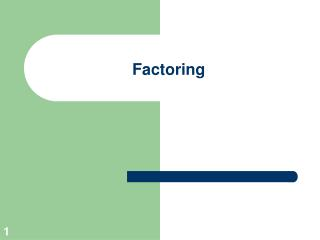DownloadDownload PresentationFactoring

# Factoring

Download Presentation## Factoring

- - - - - - - - - - - - - - - - - - - - - - - - - - - E N D - - - - - - - - - - - - - - - - - - - - - - - - - - -
##### Presentation Transcript

1. Factoring

2. Factoring—define factored form • Factor means to write a quantity as a multiplication problem • a product of the factors. • Factored forms of 18 are:

3. Factoring: The Greatest Common Factor • Factor each of the following by factoring out the greatest common factor: 5x + 5 = 4ab + 10a2 = 8p4q3 + 6p3q2 = 2y + 4y2 + 16y3=

4. Factoring Trinomials—with a coefficient of 1 for the squared term • Factor: • A trinomial will always factor as two binomials • Multiply to the last number and add to the middle: • Check using FOIL:

5. Factoring Trinomials Standard form of a quadratic trinomial Constant term Quadratic term Linear term

6. Factoring Trinomials TIP  If you study the signs of the trinomial carefully they can help you to determine the signs of the binomials Binomials have same signs They both have this sign

7. Factoring Trinomials TIP  If you study the signs of the trinomial carefully they can help you to determine the signs of the binomials Binomials have same signs They both have this sign

8. Factoring Trinomials TIP  If you study the signs of the trinomial carefully they can help you to determine the signs of the binomials Binomials have opposite signs Put larger number with this sign

9. Factoring Examples • Factor

10. Factoring Examples • Factor

11. Factoring Examples • Factor

12. Factoring Examples • Factor

13. Factoring Trinomials—primes • A PRIME POLYNOMIAL cannot be factored using only integer factors. • Factor : • The factors of 5: 1 and 5 or -1 and -5. • Since –2 cannot be obtained from them, the polynomial is prime.

14. Practice • x2 – 3x – 10 • x2 + 8x – 9 • x2 – 4x – 12 • x2 – 13x + 40 • x2 + 6x + 9 • x2 + 14x + 48

15. Class work • Pg. 181 #2 to 30 even • Pg. 185 #2 to 46even